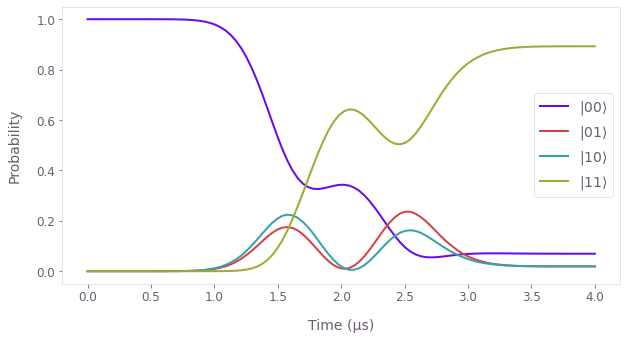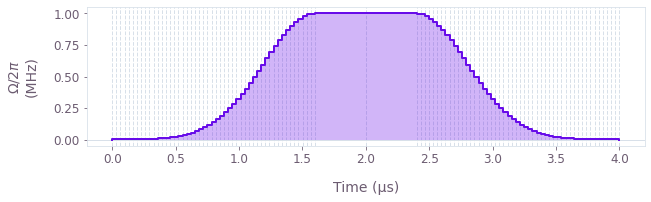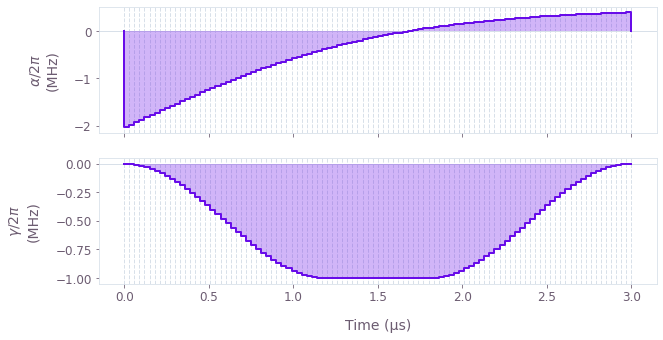•Boulder Opal
• User guides
• Create analytical signals for simulation and optimization

# How to create analytical signals for simulation and optimization

Use predefined signals from the Boulder Opal toolkit

The Boulder Opal toolkit contains analytical signals in the form of piecewise-constant functions (Pwcs), which can be used for simulation and optimization. The full list of Pwc analytical signals in the toolkit is available in the reference documentation.

The Boulder Opal Toolkits are currently in beta phase of development. Breaking changes may be introduced.

All signals require a duration, defining the time interval for the signal between zero and duration. The signals also require a segment_count, which determines the number of segments in the Pwc. Many of the signal parameters can be passed as tensors and thus can be optimized to minimize a given cost function.

In this notebook we show how to create signals from the toolkit for use in simulation and optimization. You can also define your own analytical Hamiltonians from the ground up, see this user guide for more information.

## Summary workflow

### 1. Create a node defining the signal

Once a graph has been set up for the system, create a Pwc analytic signal using one of the signals from the signal library. If one is running an optimization problem, define the optimizable signal parameters using graph.optimization_variable, before defining the signal. Then add the signal (multiplied by the appropriate operator) to the Hamiltonian.

### 2. Execute the graph for the simulation/optimization

With the graph object created, a simulation can be run using the qctrl.functions.calculate_graph function. The outputs and the graph must be provided. The function returns the results of the simulation.

Alternatively, an optimization can be run using the qctrl.functions.calculate_optimization function or the qctrl.functions.calculate_stochastic_optimization function. The cost, the outputs, and the graph must be provided. The function returns the results of the optimization.

## Example: Simulation with analytical signals

In this example, we will simulate two interacting qubits (labeled 0 and 1), with one of the qubits (qubit 0) driven by an external signal.

The Hamiltonian for the system is \begin{equation} H(t) = \frac{1}{2} \left(\Omega(t) \sigma^{-}_{0} + \Omega(t)^* \sigma^{+}_{0} \right) + \sum_{i=x,y,z} \delta_i \sigma^{i}_{0} \sigma^{i}_{1} , \end{equation} where $\Omega(t)$ is the external signal and $\delta_i$ is the qubit coupling.

The signal used for this example is the Gaussian signal, graph.signals.gaussian_pulse_pwc, defined as \begin{equation}\Omega(t) = \begin{cases} A \exp \left(- \frac{(t-t_1)^2}{2\sigma^2} \right) &\mathrm{if} \quad t < t_1=t_0- t_\mathrm{flat}/2 \\ A &\mathrm{if} \quad t_0-t_\mathrm{flat}/2 \le t < t_0+t_\mathrm{flat}/2 \\ A \exp \left(- \frac{(t-t_2)^2}{2\sigma^2} \right) &\mathrm{if} \quad t > t_2=t_0+t_\mathrm{flat}/2 \end{cases} , \end{equation} where $A$ is the amplitude, $\sigma$ is the width of the Gaussian, $t_0$ is the center of the signal, and $t_\mathrm{flat}$ is the flat duration.

import matplotlib.pyplot as plt
import numpy as np
from qctrl import Qctrl
from qctrlvisualizer import get_qctrl_style, plot_controls, plot_population_dynamics

# Start a Boulder Opal session.
qctrl = Qctrl()

plt.style.use(get_qctrl_style())
# Create the data flow graph describing the system.
graph = qctrl.create_graph()

# Define the duration of the pulse.
duration = 4e-6  # s

# Define the parameters of the pulse.
# Use a minimal segmentation for a more efficient sampling.
pulse = graph.signals.gaussian_pulse_pwc(
duration=duration,
segment_count=100,
amplitude=2 * np.pi * 1.0e6,  # rad/s
width=duration * 0.1,
flat_duration=duration * 0.2,
segmentation="MINIMAL",
name="$\\Omega$",
)

# Add the pulse terms to the Hamiltonian.
hamiltonian = graph.hermitian_part(
pulse * graph.pauli_kronecker_product([("M", 0)], subsystem_count=2)
)

# Add the qubit-qubit coupling terms to the Hamiltonian.
couplings = {"X": 8.0e6, "Y": 8.0e6, "Z": 9.0e6}  # rad/s

for i, coupling in couplings.items():
hamiltonian += coupling * graph.pauli_kronecker_product(
[(i, 0), (i, 1)], subsystem_count=2
)
sample_times = np.linspace(0.0, duration, 100)

# Compute the unitary using time_evolution_operators_pwc.
unitaries = graph.time_evolution_operators_pwc(
hamiltonian=hamiltonian, sample_times=sample_times
)

# Define the initial state.
initial_state = graph.fock_state(4, 0)[:, None]

# Evolve the initial state with the unitary to get the final state.
evolved_states = unitaries @ initial_state
evolved_states.name = "states"

# Run simulation.
result = qctrl.functions.calculate_graph(
graph=graph, output_node_names=["$\\Omega$", "states"]
)
Your task calculate_graph (action_id="1618599") has completed.

# Plot the evolution of the populations of the qubits using plot_population_dynamics.
states = result.output.pop("states")
qubit_populations = np.abs(states["value"].squeeze()) ** 2

plot_population_dynamics(
sample_times, {rf"$|{k:02b}\rangle$": qubit_populations[:, k] for k in range(4)}
)# View the generated Gaussian pulse using the plot_controls function.
plot_controls(result.output)## Example: Optimization with analytical signals

In this example, we will implement an X gate in a noisy single-qubit system, by optimizing the parameters of two signals.

The Hamiltonian for the system is \begin{equation} H(t) = \alpha(t) \sigma_{z} + \frac{1}{2}\left(\gamma(t)\sigma_{-} + \gamma^*(t)\sigma_{+}\right) + \delta \sigma_{z} + \zeta(t) \sigma_{z} , \end{equation} where $\alpha(t)$ and $\gamma(t)$ are signals with optimizable parameters, $\delta$ is the qubit detuning, and $\zeta(t)$ is a dephasing noise process.

The $\alpha(t)$ signal acting on $\sigma_{z}$ is a hyperbolic tangent ramp, graph.signals.tanh_ramp_pwc, defined by \begin{equation} \alpha(t) = \frac{a_+ + a_-}{2} + \frac{a_+ - a_-}{2} \tanh\left( \frac{t - t_0}{t_\mathrm{ramp}} \right) , \end{equation} where $a_\pm$ are the asymptotic values of the signal, $t_0$ is the center time, and $t_\mathrm{ramp}$ is the characteristic duration of the ramp.

The $\gamma(t)$ signal acting on $\sigma_{-}$ is a cosine pulse, graph.signals.cosine_pulse_pwc, defined by \begin{equation} \gamma(t) = \frac{A}{2} \left[1 + \cos \left(\frac{2\pi}{\tau} t- \pi \right)\right] = A \sin^2 \left(\frac{\pi}{\tau}t\right) , \end{equation} where $A$ is the amplitude and $\tau$ is the given duration of the signal.

graph = qctrl.create_graph()

# Define the duration of the signals.
duration = 3e-6  # s

# Define the number of segments for Pwc signals.
segment_count = 100

# Define α tanh ramp acting on sigma_z.
alpha_start_value = graph.optimization_variable(
count=1, lower_bound=-3e7, upper_bound=3e7, name="alpha_start_value"
)
alpha_end_value = graph.optimization_variable(
count=1, lower_bound=-3e7, upper_bound=3e7, name="alpha_end_value"
)
alpha_ramp_duration = graph.optimization_variable(
count=1, lower_bound=0.1e-6, upper_bound=duration, name="alpha_ramp_duration"
)
alpha_center_time = graph.optimization_variable(
count=1, lower_bound=0.0, upper_bound=duration, name="alpha_center_time"
)
alpha_ramp = graph.signals.tanh_ramp_pwc(
duration=duration,
segment_count=segment_count,
start_value=alpha_start_value,
end_value=alpha_end_value,
ramp_duration=alpha_ramp_duration,
center_time=alpha_center_time,
name=r"$\alpha$",
)

# Define γ cosine pulse acting on sigma_-.
gamma_amplitude = graph.optimization_variable(
count=1,
lower_bound=-2.0 * np.pi * 1e6,  # rad/s
upper_bound=2.0 * np.pi * 1e6,  # rad/s
name="gamma_amplitude",
)
gamma_pulse = graph.signals.cosine_pulse_pwc(
duration=duration,
segment_count=segment_count,
amplitude=gamma_amplitude,
flat_duration=duration * 0.2,
name=r"$\gamma$",
)

# Create pulse Hamiltonian.
hamiltonian = alpha_ramp * graph.pauli_matrix("Z")
hamiltonian += graph.hermitian_part(gamma_pulse * graph.pauli_matrix("M"))

delta = 2 * np.pi * 0.25e6  # rad/s
hamiltonian += delta * graph.pauli_matrix("Z")

# Define target gate.
target = graph.target(operator=graph.pauli_matrix("X"))

zeta = 2 * np.pi * 20e3  # rad/s
dephasing = zeta * graph.pauli_matrix("Z")

# Robust infidelity.
robust_infidelity = graph.infidelity_pwc(
hamiltonian=hamiltonian,
noise_operators=[dephasing],
target=target,
name="robust_infidelity",
)

# Compute the unitary using time_evolution_operators_pwc.
unitary = graph.time_evolution_operators_pwc(
hamiltonian=hamiltonian, sample_times=np.array([duration]), name="unitary"
)

result = qctrl.functions.calculate_optimization(
graph=graph,
cost_node_name="robust_infidelity",
output_node_names=[
"alpha_start_value",
"alpha_end_value",
"alpha_ramp_duration",
"alpha_center_time",
"gamma_amplitude",
"unitary",
r"$\alpha$",
r"$\gamma$",
],
optimization_count=8,
)

print(f"Optimized cost: {result.cost:.3e}")
Your task calculate_optimization (action_id="1618602") has started.
Optimized cost: 7.463e-04

# Check that the unitary operator associated with the evolution of the system Hamiltonian
# does indeed correspond to an X gate.
result.output.pop("unitary")["value"].round(5)
array([[[-4.09e-03+1.9000e-04j, -2.00e-05+9.9999e-01j],
[ 2.00e-05+9.9999e-01j, -4.09e-03-1.9000e-04j]]])

# Print out the converged signal parameters.
print("Alpha signal")
for param in ["start_value", "end_value"]:

for param in ["ramp_duration", "center_time"]:
print(f"\t{param}: \t{result.output.pop(f'alpha_{param}')['value']:.3e} s")

print("Gamma signal")
for param in ["amplitude"]:
print(f"\t{param}: \t{result.output.pop(f'gamma_{param}')['value']:.3e} rad/s")
Alpha signal
ramp_duration: 	1.488e-06 s
center_time: 	4.483e-08 s
Gamma signal

# View the optimized signals using the plot_controls function.
plot_controls(result.output)This notebook was run using the following package versions. It should also be compatible with newer versions of the Q-CTRL Python package.

PackageVersion
Python3.10.8
matplotlib3.6.3
numpy1.23.1
scipy1.10.0
qctrl20.2.0
qctrl-commons17.10.0
boulder-opal-toolkits2.0.0-beta.4
qctrl-visualizer4.6.0